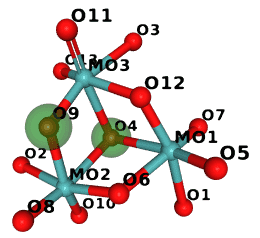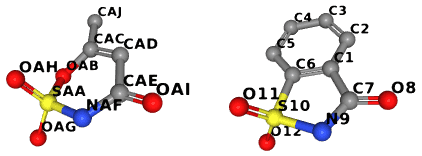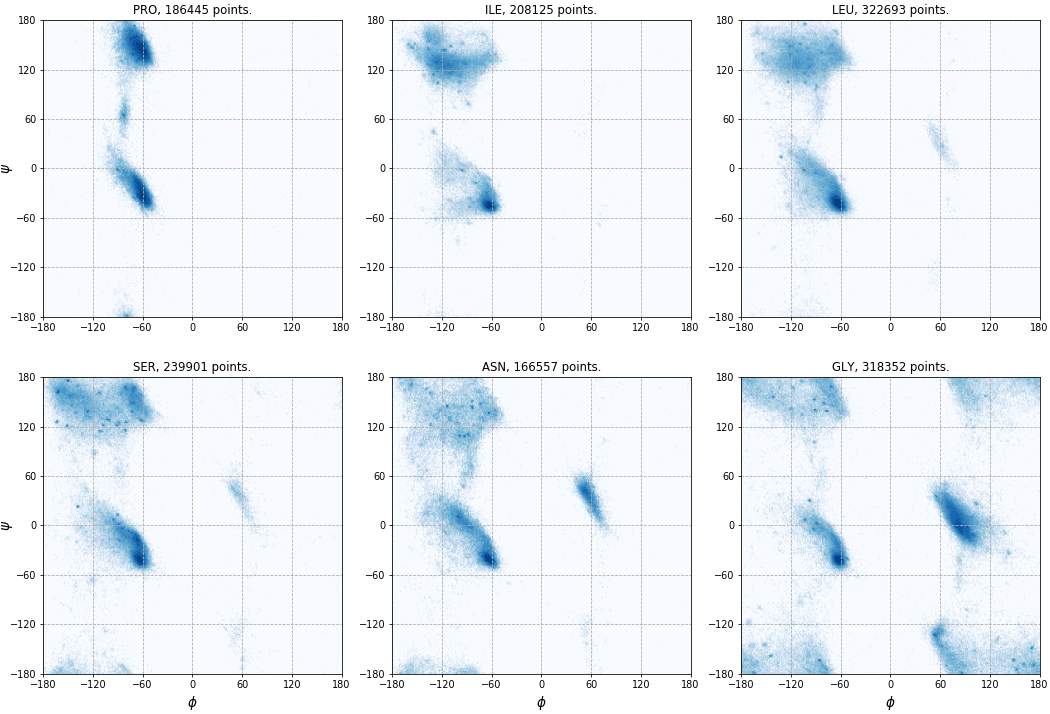# Structure analysis¶

## Selections¶

For now, Gemmi supports only the selection syntax from MMDB, called CID (Coordinate ID). The syntax is described at the bottom of the pdbcur documentation.

The selections in Gemmi are not widely used yet and the API may evolve. The examples below demonstrates currently provided functions.

Example 1

Working with CID selections.

```>>> st = gemmi.read_structure('../tests/1pfe.cif.gz')

>>> # select all Cl atoms
>>> sel = gemmi.parse_cid('[CL]')
>>> # get the first result as pointer to model and CRA (chain, residue, atom)
>>> sel.first(st)
(<gemmi.Model 1 with 2 chain(s)>, <gemmi.CRA A/CL 20/CL>)

>>> sel = gemmi.parse_cid('A/1-4/N9')
>>> sel.to_cid()
'//A/1.-4./N9'
>>> # iterate over hierarchy filtered by the selection
>>> for model in sel.models(st):
...     for chain in sel.chains(model):
...         print('-', chain.name)
...         for residue in sel.residues(chain):
...             print('   -', str(residue))
...             for atom in sel.atoms(residue):
...                 print('          -', atom.name)
...
- A
- 1(DG)
- N9
- 2(DC)
- 3(DG)
- N9
- 4(DT)
```

Example 2

Copy alpha-carbon atoms to a new structure (or a model).

```>>> st = gemmi.read_structure('../tests/1orc.pdb')
>>> st.count_atom_sites()
559
>>> selection = gemmi.parse_cid('CA[C]')

>>> # create a new structure
>>> ca_st = selection.copy_structure_selection(st)
>>> ca_st.count_atom_sites()
64

>>> # create a new model
>>> ca_model = selection.copy_model_selection(st)
>>> ca_model.count_atom_sites()
64
```

Example 3

Select residues in the radius of 8Å from a selected point.

```>>> selected_point = gemmi.Position(20, 40, 30)
>>> ns = gemmi.NeighborSearch(st, st.cell, 8.0).populate()
>>> for mark in ns.find_atoms(selected_point):
...     mark.to_cra(st).residue.flag = 's'
>>> selection = gemmi.Selection().set_residue_flags('s')
>>> selection.copy_model_selection(st).count_atom_sites()
121
```

Note: NeighborSearch searches for atoms in all symmetry images. This is why it takes UnitCell as a parameter. To search only in atoms directly listed in the file pass empty cell (`gemmi.UnitCell()`).

Example 3a

Select atoms in the radius of 8Å from a selected point.

```>>> # selected_point and ns are reused from the previous example
>>> for mark in ns.find_atoms(selected_point):
...     mark.to_cra(st).atom.flag = 's'
>>> selection = gemmi.Selection().set_atom_flags('s')
>>> selection.copy_model_selection(st).count_atom_sites()
59
```

## Graph analysis¶

The graph algorithms in Gemmi are limited to finding the shortest path between atoms (bonds = graph edges). This part of the library is not documented yet.

The rest of this section shows how to use Gemmi together with external graph analysis libraries to analyse the similarity of chemical molecules. To do this, first we set up a graph corresponding to the molecule.

Here we show how it can be done in the Boost Graph Library.

```#include <boost/graph/graph_traits.hpp>
#include <gemmi/chemcomp.hpp>        // for ChemComp, make_chemcomp_from_block

struct AtomVertex {
gemmi::Element el = gemmi::El::X;
std::string name;
};

struct BondEdge {
gemmi::BondType type;
};

boost::undirectedS,
AtomVertex, BondEdge>;

Graph make_graph(const gemmi::ChemComp& cc) {
Graph g(cc.atoms.size());
for (size_t i = 0; i != cc.atoms.size(); ++i) {
g[i].el = cc.atoms[i].el;
g[i].name = cc.atoms[i].id;
}
for (const gemmi::Restraints::Bond& bond : cc.rt.bonds) {
int n1 = cc.get_atom_index(bond.id1.atom);
int n2 = cc.get_atom_index(bond.id2.atom);
}
return g;
}
```

And here we use NetworkX in Python:

```>>> import networkx

>>> G = networkx.Graph()
>>> so3 = gemmi.make_chemcomp_from_block(block)
>>> for atom in so3.atoms:
...
>>> for bond in so3.rt.bonds:
...     G.add_edge(bond.id1.atom, bond.id2.atom)  # ignoring bond type
...
```

To show a quick example, let us count automorphisms of SO3:

```>>> import networkx.algorithms.isomorphism as iso
>>> GM = iso.GraphMatcher(G, G, node_match=iso.categorical_node_match('Z', 0))
>>> # expecting 3! automorphisms (permutations of the three oxygens)
>>> sum(1 for _ in GM.isomorphisms_iter())
6
```

With a bit more of code we could perform a real cheminformatics task.

### Graph isomorphism¶

In this example we use Python NetworkX to compare molecules from the Refmac monomer library with Chemical Component Dictionary (CCD) from PDB. The same could be done with other graph analysis libraries, such as Boost Graph Library, igraph, etc.

The program below takes compares specified monomer cif files with corresponding CCD entries. Hydrogens and bond types are ignored. It takes less than half a minute to go through the 25,000 monomer files distributed with CCP4 (as of Oct 2018), so we do not try to optimize the program.

```# Compares graphs of molecules from cif files (Refmac dictionary or similar)
# with CCD entries.

import sys
import networkx
from networkx.algorithms import isomorphism
import gemmi

CCD_PATH = 'components.cif.gz'

def graph_from_chemcomp(cc):
G = networkx.Graph()
for atom in cc.atoms:
for bond in cc.rt.bonds:
return G

def compare(cc1, cc2):
s1 = {a.id for a in cc1.atoms}
s2 = {a.id for a in cc2.atoms}
b1 = {b.lexicographic_str() for b in cc1.rt.bonds}
b2 = {b.lexicographic_str() for b in cc2.rt.bonds}
if s1 == s2 and b1 == b2:
#print(cc1.name, "the same")
return
G1 = graph_from_chemcomp(cc1)
G2 = graph_from_chemcomp(cc2)
node_match = isomorphism.categorical_node_match('Z', 0)
GM = isomorphism.GraphMatcher(G1, G2, node_match=node_match)
if GM.is_isomorphic():
print(cc1.name, 'is isomorphic')
# we could use GM.match(), but here we try to find the shortest diff
short_diff = None
for n, mapping in enumerate(GM.isomorphisms_iter()):
diff = {k: v for k, v in mapping.items() if k != v}
if short_diff is None or len(diff) < len(short_diff):
short_diff = diff
if n == 10000:  # don't spend too much here
print(' (it may not be the simplest isomorphism)')
break
for id1, id2 in short_diff.items():
print('\t', id1, '->', id2)
else:
print(cc1.name, 'differs')
if s2 - s1:
print('\tmissing:', ' '.join(s2 - s1))
if s1 - s2:
print('\textra:  ', ' '.join(s1 - s2))

def main():
absent = 0
for f in sys.argv[1:]:
cc1 = gemmi.make_chemcomp_from_block(block)
try:
block2 = ccd[cc1.name]
except KeyError:
absent += 1
#print(cc1.name, 'not in CCD')
continue
cc2 = gemmi.make_chemcomp_from_block(block2)
cc1.remove_hydrogens()
cc2.remove_hydrogens()
compare(cc1, cc2)
if absent != 0:

main()
```

If we run it on monomers that start with M we get:

```\$ examples/ccd_gi.py \$CLIBD_MON/m/*.cif
M10 is isomorphic
O9 -> O4
O4 -> O9
MK8 is isomorphic
O2 -> OXT
MMR differs
missing: O12 O4
```

So in M10 the two atoms marked green are swapped:(The image was generated in NGL and compressed with Compress-Or-Die.)

### Substructure matching¶

Now a little script to illustrate subgraph isomorphism. The script takes a (three-letter-)code of a molecule that is to be used as a pattern and finds CCD entries that contain such a a substructure. As in the previous example, hydrogens and bond types are ignored.

```# List CCD entries that contain the specified entry as a substructure.
# Ignoring hydrogens and bond types.

import sys
import networkx
from networkx.algorithms import isomorphism
import gemmi

CCD_PATH = 'components.cif.gz'

def graph_from_block(block):
cc = gemmi.make_chemcomp_from_block(block)
cc.remove_hydrogens()
G = networkx.Graph()
for atom in cc.atoms:
for bond in cc.rt.bonds:
return G

def main():
assert len(sys.argv) == 2, "Usage: ccd_subgraph.py three-letter-code"
entry = sys.argv
pattern = graph_from_block(ccd[entry])
pattern_nodes = networkx.number_of_nodes(pattern)
pattern_edges = networkx.number_of_edges(pattern)
node_match = isomorphism.categorical_node_match('Z', 0)
for block in ccd:
G = graph_from_block(block)
GM = isomorphism.GraphMatcher(G, pattern, node_match=node_match)
if GM.subgraph_is_isomorphic():
print(block.name, '\t +%d nodes, +%d edges' % (
networkx.number_of_nodes(G) - pattern_nodes,
networkx.number_of_edges(G) - pattern_edges))

main()
```

Let us check what entries have HEM as a substructure:

```\$ examples/ccd_subgraph.py HEM
1FH    +6 nodes, +7 edges
2FH    +6 nodes, +7 edges
4HE    +7 nodes, +8 edges
522    +2 nodes, +2 edges
6CO    +6 nodes, +7 edges
6CQ    +7 nodes, +8 edges
89R    +3 nodes, +3 edges
CLN    +1 nodes, +2 edges
DDH    +2 nodes, +2 edges
FEC    +6 nodes, +6 edges
HAS    +22 nodes, +22 edges
HCO    +1 nodes, +1 edges
HDM    +2 nodes, +2 edges
HEA    +17 nodes, +17 edges
HEB    +0 nodes, +0 edges
HEC    +0 nodes, +0 edges
HEM    +0 nodes, +0 edges
HEO    +16 nodes, +16 edges
HEV    +2 nodes, +2 edges
HP5    +2 nodes, +2 edges
ISW    +0 nodes, +0 edges
MH0    +0 nodes, +0 edges
MHM    +0 nodes, +0 edges
N7H    +3 nodes, +3 edges
NTE    +3 nodes, +3 edges
OBV    +14 nodes, +14 edges
SRM    +20 nodes, +20 edges
UFE    +18 nodes, +18 edges
```

### Maximum common subgraph¶

In this example we use McGregor’s algorithm implemented in the Boost Graph Library to find maximum common induced subgraph. We call the MCS searching function with option `only_connected_subgraphs=true`, which has obvious meaning and can be changed if needed.

To illustrate this example, we compare ligands AUD and LSA:The whole code is in `examples/with_bgl.cpp`. The same file has also examples of using the BGL implementation of VF2 to check graph and subgraph isomorphisms.

```// We ignore hydrogens here.
// Example output:
//   \$ time with_bgl -c monomers/a/AUD.cif monomers/l/LSA.cif
//   Searching largest subgraphs of AUD and LSA (10 and 12 vertices)...
//   Maximum connected common subgraph has 7 vertices:
//     SAA -> S10
//     OAG -> O12
//     OAH -> O11
//     NAF -> N9
//     CAE -> C7
//     OAI -> O8
//   Maximum connected common subgraph has 7 vertices:
//     SAA -> S10
//     OAG -> O11
//     OAH -> O12
//     NAF -> N9
//     CAE -> C7
//     OAI -> O8
//
//   real	0m0.012s
//   user	0m0.008s
//   sys	0m0.004s

struct PrintCommonSubgraphCallback {
Graph g1, g2;
template <typename CorrespondenceMap1To2, typename CorrespondenceMap2To1>
bool operator()(CorrespondenceMap1To2 map1, CorrespondenceMap2To1,
typename GraphTraits::vertices_size_type subgraph_size) {
std::cout << "Maximum connected common subgraph has " << subgraph_size
<< " vertices:\n";
for (auto vp = boost::vertices(g1); vp.first != vp.second; ++vp.first) {
Vertex v1 = *vp.first;
Vertex v2 = boost::get(map1, v1);
if (v2 != GraphTraits::null_vertex())
std::cout << "  " << g1[v1].name << " -> " << g2[v2].name << std::endl;
}
return true;
}
};

void find_common_subgraph(const char* cif1, const char* cif2) {
gemmi::ChemComp cc1 = make_chemcomp(cif1).remove_hydrogens();
Graph graph1 = make_graph(cc1);
gemmi::ChemComp cc2 = make_chemcomp(cif2).remove_hydrogens();
Graph graph2 = make_graph(cc2);
std::cout << "Searching largest subgraphs of " << cc1.name << " and "
<< cc2.name << " (" << cc1.atoms.size() << " and " << cc2.atoms.size()
<< " vertices)..." << std::endl;
mcgregor_common_subgraphs_maximum_unique(
graph1, graph2,
get(boost::vertex_index, graph1), get(boost::vertex_index, graph2),
[&](Edge a, Edge b) { return graph1[a].type == graph2[b].type; },
[&](Vertex a, Vertex b) { return graph1[a].el == graph2[b].el; },
/*only_connected_subgraphs=*/ true,
PrintCommonSubgraphCallback{graph1, graph2});
}
```

## Torsion Angles¶

This section presents functions dedicated to calculation of the dihedral angles φ (phi), ψ (psi) and ω (omega) of the protein backbone. These functions are built upon the more general `calculate_dihedral` function, introduced in the section about coordinates, which takes four points in the space as arguments.

`calculate_omega()` calculates the ω angle, which is usually around 180°:

```>>> from math import degrees
>>> degrees(gemmi.calculate_omega(chain, chain))
159.90922150065668
>>> for res in chain[:5]:
...     next_res = chain.next_residue(res)
...     if next_res:
...         omega = gemmi.calculate_omega(res, next_res)
...         print(res.name, degrees(omega))
...
ALA 159.90922150065668
ALA -165.26874513591105
ALA -165.85686681169656
THR -172.99968385093513
SER 176.74223937657646
```

The φ and ψ angles are often used together, so they are calculated in one function `calculate_phi_psi()`:

```>>> for res in chain[:5]:
...     prev_res = chain.previous_residue(res)
...     next_res = chain.next_residue(res)
...     phi, psi = gemmi.calculate_phi_psi(prev_res, res, next_res)
...     print('%s %8.2f %8.2f' % (res.name, degrees(phi), degrees(psi)))
...
ALA      nan   106.94
ALA  -116.64    84.57
ALA   -45.57   127.40
THR   -62.01   147.45
SER   -92.85   161.53
```

In C++ these functions can be found in `gemmi/calculate.hpp`.

The torsion angles φ and ψ can be visualized on the Ramachandran plot. Let us plot angles from all PDB entries with the resolution higher than 1.5A. Usually, glycine, proline and the residue preceding proline (pre-proline) are plotted separately. Here, we will exclude pre-proline and make separate plot for each amino acid. So first, we calculate angles and save φ,ψ pairs in a set of files – one file per residue.

```import sys
from math import degrees
import gemmi

ramas = {aa: [] for aa in [
'LEU', 'ALA', 'GLY', 'VAL', 'GLU', 'SER', 'LYS', 'ASP', 'THR', 'ILE',
'ARG', 'PRO', 'ASN', 'PHE', 'GLN', 'TYR', 'HIS', 'MET', 'CYS', 'TRP']}

for path in gemmi.CoorFileWalk(sys.argv):
if 0.1 < st.resolution < 1.5:
model = st
for chain in model:
for res in chain.get_polymer():
# previous_residue() and next_residue() return previous/next
# residue only if the residues are bonded. Otherwise -- None.
prev_res = chain.previous_residue(res)
next_res = chain.next_residue(res)
if prev_res and next_res and next_res.name != 'PRO':
v = gemmi.calculate_phi_psi(prev_res, res, next_res)
try:
ramas[res.name].append(v)
except KeyError:
pass

# Write data to files
for aa, data in ramas.items():
with open('ramas/' + aa + '.tsv', 'w') as f:
for phi, psi in data:
f.write('%.4f\t%.4f\n' % (degrees(phi), degrees(psi)))
```

The script above works with coordinate files in any of the formats supported by gemmi (PDB, mmCIF, mmJSON). As of 2019, processing a local copy of the PDB archive in the PDB format takes about 20 minutes.

In the second step we plot the data points with matplotlib. We use a script that can be found in `examples/rama_gather.py`. Six of the resulting plots are shown here (click to enlarge):## Local copy of the PDB archive¶

Some examples in this documentation work on a local copy of the Protein Data Bank archive. This subsection is actually a footnote describing our setup.

Like in BioJava, we assume that the `\$PDB_DIR` environment variable points to a directory that contains `structures/divided/mmCIF` – the same arrangement as on the PDB’s FTP server.

```\$ cd \$PDB_DIR
\$ du -sh structures/*/*  # as of Jun 2017
34G    structures/divided/mmCIF
25G    structures/divided/pdb
101G   structures/divided/structure_factors
2.6G   structures/obsolete/mmCIF
```

A traditional way to keep an up-to-date local archive is to rsync it once a week:

```#!/bin/sh -x
set -u  # PDB_DIR must be defined
rsync_subdir() {
mkdir -p "\$PDB_DIR/\$1"
# Using PDBe (UK) here, can be replaced with RCSB (USA) or PDBj (Japan),